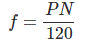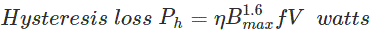# Types of Losses in DC Machines

Posted on

Losses in DC machine is divided in to Copper Losses and Stray Losses. Stray Losses further divided in to Iron Losses and Mechanical Losses. Mechanical Loss consists of Friction Loss and Windage Loss. And it is 10 to 20 percentage of full Load Losses. Iron Loss is 20 to 30 percent and called Core Loss. And it is a Magnetic Loss.

It can be further divided in to Hysteresis Loss and Eddy Current Loss. Copper Loss is due to resistances. It can be of armature resistance or field resistance or brush contact resistances. Field Copper Loss is 20 to 30 % of full Load Copper Losses and can be shunt or series. Shunt field Copper Loss and stay Losses marked in yellow are constant loss. Armature Copper Loss is a variable loss.

# Types of Losses in DC Machines

As we know “Energy neither can be created nor it can be destroyed, it can only be transferred from one form to another”. In DC machine, mechanical energy is converted into the electrical energy.

During this process, the total input power is not transformed into output power. Some part of input power gets wasted in various forms. The form of this loss may vary from one machine to another.

These losses give in the rise in temperature of the machine and reduce the efficiency of the machine. In DC Machine, there are broadly four main categories of energy loss.

The losses can be divided into three types in a dc machine (Generator or Motor). They are

1. Copper losses
2. Iron or Core losses and
3. Mechanical losses.
All these losses seem as heat and therefore increase the temperature of the machine. Further the efficiency of the machine will reduce. Now we will discuss types of losses in dc machines one by one for better understanding the subject.

## Copper Losses

The copper losses are the winding losses taking place during the current flowing through the winding. These losses occur due to the resistance in the winding. In a DC machine, there are only two windings, armature and field winding.

Thus copper losses categories in three parts; armature loss, the field winding loss, and brush contact resistance loss. The copper losses are proportional to the square of the current flowing through the winding.

### Armature Copper Loss

Armature copper loss = Ia2Ra

Where,

• Ia is armature current and
• Ra is armature resistance.

These losses are about 30% of the total full load losses.

### Field Winding Copper Loss

Field winding copper loss = If2Rf

Where,

• If is field current and
• Rf is field resistance.

These losses are about 25% theoretically, but practically it is constant.

### Brush Contact Resistance Loss

Brush contact loss attributes to resistance between the surface of brush and commutator. It is not a loss which could be calculated separately as it is a part of variable losses. Generally, it contributes to both the types of copper losses. So, they are factors in the calculation of the above losses.

## Iron or Core Losses

As iron core of the armature is rotating in magnetic field, some losses occurs in the core which is called core losses. Normally, machines are operated with constant speed, so these losses are almost constant. These losses are categorized in two form; Hysteresis loss and Eddy current loss.

### Hysteresis Loss

Hysteresis losses occur in the armature winding due to reversal of magnetization of the core. When the core of the armature exposed to the magnetic field, it undergoes one complete rotation of magnetic reversal.

The portion of the armature which is under S-pole, after completing half electrical revolution, the same piece will be under the N-pole, and the magnetic lines are reversed in order to overturn the magnetism within the core.

The constant process of magnetic reversal in the armature, consume some amount of energy which is called hysteresis loss. The percentage of loss depends upon the quality and volume of the iron.

#### The Frequency of Magnetic ReversalWhere,

• P = Number of poles
• N = Speed in rpm

#### Steinmetz Formula

The Steinmetz formula is for the calculation of hysteresis loss.Where,

• η = Steinmetz hysteresis co-efficient
• Bmax = Maximum flux Density in armature winding
• F = Frequency of magnetic reversals
• V = Volume of armature in m3.

### Eddy Current Loss

According to Faraday’s law of electromagnetic induction, when an iron core rotates in the magnetic field, an emf is also induced in the core. Similarly, when armature rotates in the magnetic field, the small amount of emf induced in the core which allows the flow of charge in the body due to the conductivity of the core.

This current is useless for the machine. This loss of current is called eddy current. This loss is almost constant for the DC machines. It could be minimized by selecting the laminated core.

## Mechanical Losses

The losses associated with mechanical friction of the machine are called mechanical losses. These losses occur due to friction in the moving parts of the machine like bearing, brushes etc, and windage losses occur due to the air inside the rotating coil of the machine. These losses are usually very small about 15% of full load loss.

## Stray Load Losses

There are some more losses other than the losses which have been discussed above. These losses are called stray-load losses. These miscellaneous losses are due to the short-circuit current in the coil undergoing commutation, distortion of flux due to the armature and many more losses which are difficult to find. These losses are difficult to determine. However, they are taken as 1% of the whole load power output.

### Types of Losses in DC Machines Conclusion

After going through the above portion of losses in dc machine we can now establish types of losses in dc machines. I hope you enjoy when reading this article, thank you.